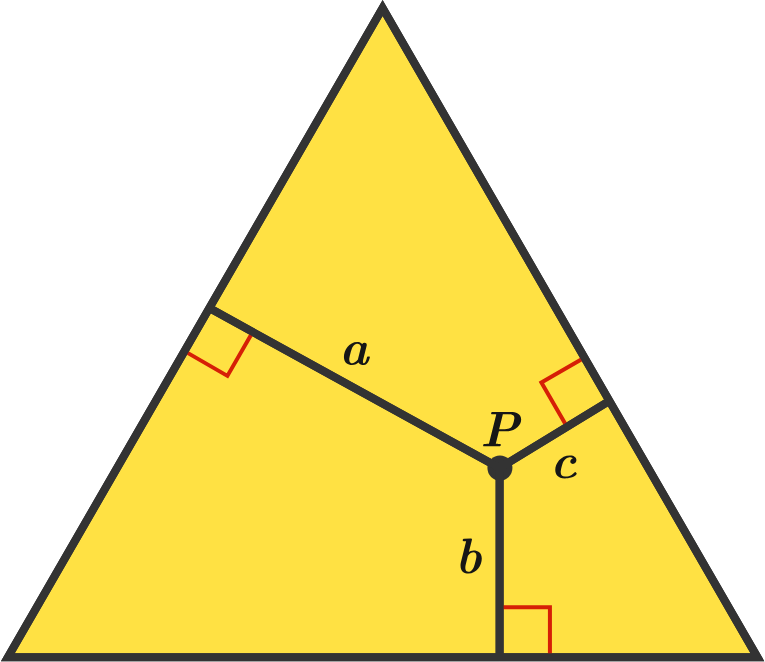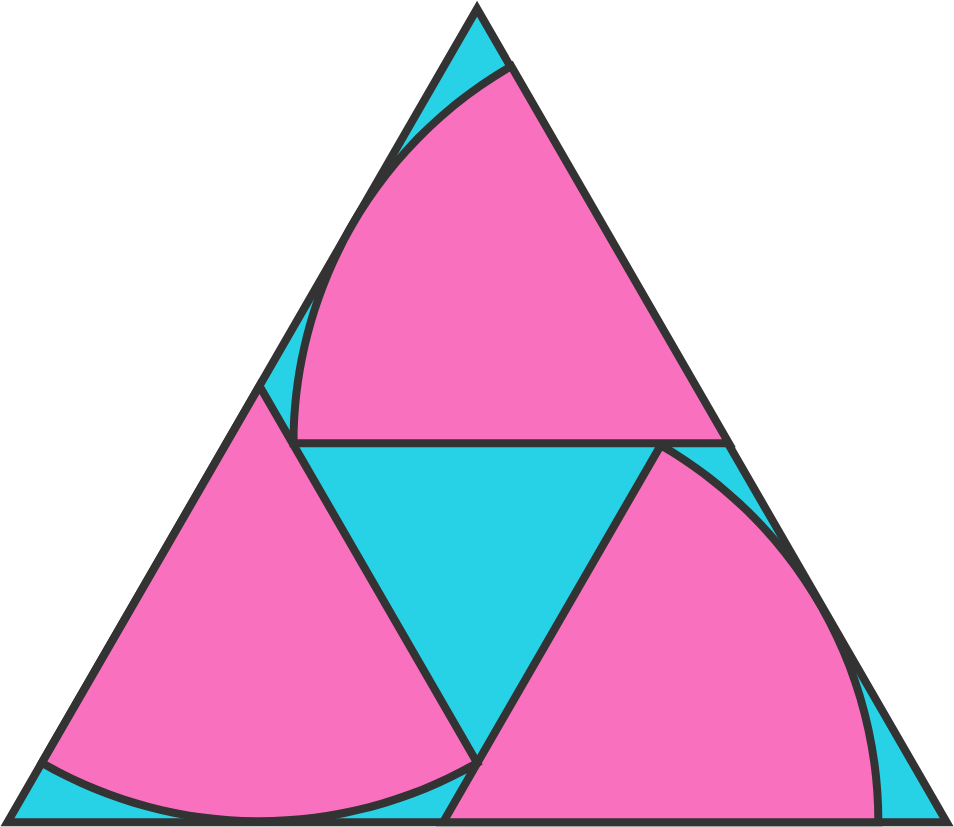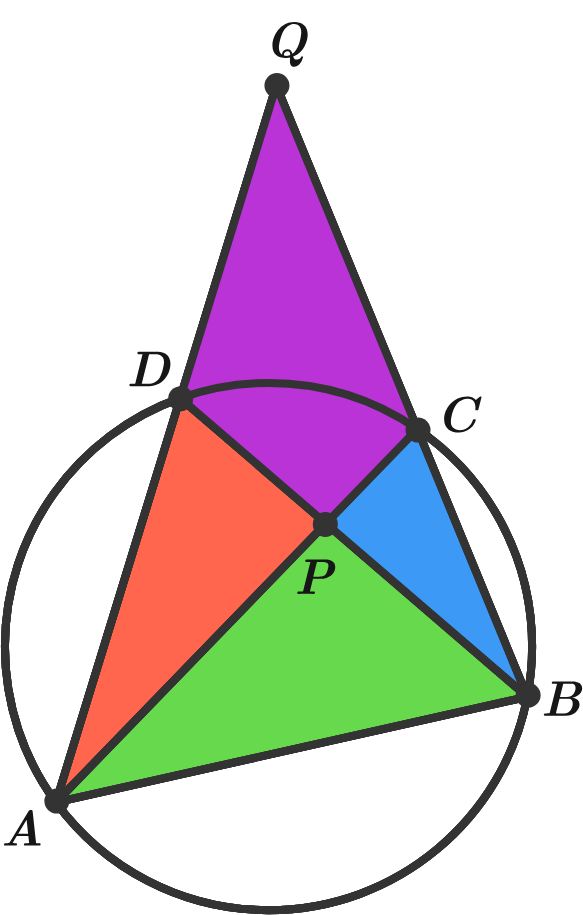# Problems of the Week

Contribute a problem

# 2018-10-15 IntermediateTrue or False?

The sum $a+b+c$ varies depending on the location of $P.$

Clarification: Point $P$ lies inside of this equilateral triangle, at perpendicular distances $a$, $b$, and $c$ from the sides of the triangle.

True or False?

If $a,b,c,$ and $n$ are positive integers such that $n=a^2+b^2+c^2,$ then there exist positive integers $d,e,$ and $f$ such that $n^2=d^2+e^2+f^2.$

In other words, if $n$ is the sum of three squares of positive integers, then $n^2$ is also the sum of three squares of positive integers.Three $60^{\circ}$-sectors of a unit circle pack neatly inside an equilateral triangle.

The side length of this triangle can be written as $A+\frac{B}{\sqrt{C}},$ where $A, B, C$ are integers with $C$ square-free.

What is the value of $A+B+C?$Twenty people at a convention are of different ages and have different names.

The following is true of four of the people:

• Allen is older than Bob.
• Bob is older than Carrie.
• Bob is older than Dave.

If Edward is part of this convention, the probability that Dave is older than Edward is $\frac{a}{b},$ where $a$ and $b$ are coprime positive integers.

What is $a+b?$

Assume that for any particular age, each person has the same chance to be that age.In the diagram, $A, B, C,$ and $D$ are concyclic points.

What is the area of the green triangle $APB,$ given the areas of the three other colored regions?

\begin{aligned} \color{#D61F06}S_{\Delta APD}&=27\\ \color{#69047E}S_{CPDQ}&=37\\ \color{#3D99F6}S_{\Delta BPC}&=12\\ \color{#20A900}S_{\Delta APB}&=\ ? \end{aligned}

Bonus: The four points need not to be concyclic; solve it without this information.

×